Test: Chemical Equilibrium - 1

# Test: Chemical Equilibrium - 1

Test Description

## 20 Questions MCQ Test Physical Chemistry | Test: Chemical Equilibrium - 1

Test: Chemical Equilibrium - 1 for Chemistry 2022 is part of Physical Chemistry preparation. The Test: Chemical Equilibrium - 1 questions and answers have been prepared according to the Chemistry exam syllabus.The Test: Chemical Equilibrium - 1 MCQs are made for Chemistry 2022 Exam. Find important definitions, questions, notes, meanings, examples, exercises, MCQs and online tests for Test: Chemical Equilibrium - 1 below.
Solutions of Test: Chemical Equilibrium - 1 questions in English are available as part of our Physical Chemistry for Chemistry & Test: Chemical Equilibrium - 1 solutions in Hindi for Physical Chemistry course. Download more important topics, notes, lectures and mock test series for Chemistry Exam by signing up for free. Attempt Test: Chemical Equilibrium - 1 | 20 questions in 60 minutes | Mock test for Chemistry preparation | Free important questions MCQ to study Physical Chemistry for Chemistry Exam | Download free PDF with solutions
 1 Crore+ students have signed up on EduRev. Have you?
Test: Chemical Equilibrium - 1 - Question 1

### For the pair of reactions given below, i)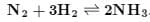(ii) 1/2N2(g) + 3/2H2(g) ⇔ NH3(g) If at a particular temperature, Kp1 and Kp2 are the equilibrium constants for reaction (i) and (ii) respectively then,

Detailed Solution for Test: Chemical Equilibrium - 1 - Question 1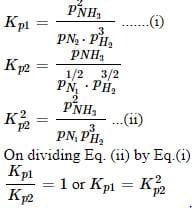Test: Chemical Equilibrium - 1 - Question 2

### Among the following, the equilibrium which is not affected by an increase in pressure is:

Detailed Solution for Test: Chemical Equilibrium - 1 - Question 2

As several moles of gaseous species are equal on both sides. Hence, for option (D), equilibrium is not affected by an increase in pressure.

Test: Chemical Equilibrium - 1 - Question 3

### Under the equilibrium condition for the reaction,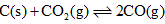the total pressure is 12 atm. If 50% of CO2 reacts then value of Kp is:

Test: Chemical Equilibrium - 1 - Question 4

For the reaction,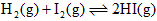the equilibrium constant Kp changes with:

Test: Chemical Equilibrium - 1 - Question 5

Pure ammonia is placed in a vessel at a temperature where its dissociation constant (α) is appreciable. At equilibrium,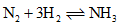Test: Chemical Equilibrium - 1 - Question 6

One mole of N2O4(g) at 300 K is kept in a closed container under one atmosphere. It is heated to 600 K when 20% by mass of N2O4(g) decomposes to NO2(g). The resultant pressure is:

Detailed Solution for Test: Chemical Equilibrium - 1 - Question 6

N2O4 → 2NO2

moles of unreacted N2O4 = 1 (1 - 0.2) = 0.8

moles of NO2 = 2 * 0.2 = 0.4
- - - - - - - - - - - - - - - - - - - - - - - - - - - - - - - - (+)
total moles n2 = 0.8 + 0.4 = 1.2

P1/(T1 n1) = P2/(T2 * n2)

1/(300 * 1) = P2/(600 * 1.2)

P2 = 2.4 atm

Test: Chemical Equilibrium - 1 - Question 7

For the reaction: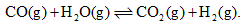At a given temperature, the equilibrium amount of CO2(g) can be increased by.

Test: Chemical Equilibrium - 1 - Question 8

For the chemical reaction,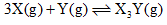The amount of X3 Y at equilibrium is affected by

Detailed Solution for Test: Chemical Equilibrium - 1 - Question 8

There are 3 main factors that affect the equilibrium of a reaction:

• Concentration of reactants or products
• Temperature
• Pressure (Affects only gaseous reactions)
Test: Chemical Equilibrium - 1 - Question 9

pH of a saturated solution of Ba(OH)2 is 12. The value of solubility product (Ksp) of Ba(OH)2 is

Detailed Solution for Test: Chemical Equilibrium - 1 - Question 9

To find out Ksp we need to know solubility of OH- which we can calculate in this case by using the value of pH.

pH+pOH=14

pOH=14-12 = 2

[OH-]=102

The following equilibrium is established when Barium hyfroxide is dissolved.

=  s   +    2s

it'sthe 2s which is equal to 10-2

so    s = 5 * 10-3

the solubility product (Ksp) of Barium Hydroxide is 4s3

so putting 102 to 4s3  we get 5 * 10-7.

Test: Chemical Equilibrium - 1 - Question 10

When two reactants, A and B are mixed to give products, C and D, the reaction quotient, (Q) at the initial stages of the reaction:

Detailed Solution for Test: Chemical Equilibrium - 1 - Question 10

Given reaction is A+B⇌C+D
The  reaction quotient Q is written by multiplying concentrations of products and dividing by concentration products of reactants

Q = [C][D]/[A][B]

At initial stages of reaction, formation of products C and D takes place; i.e, the concentration od C & D increases at the expenses of A & B concentration, which decreases.
∴Q increases with respect to time

Test: Chemical Equilibrium - 1 - Question 11

At constant temperature, the equilibrium (Kp) for the decomposition reaction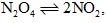is expressed by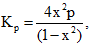where, p = pressure,

X = extent of decomposition. Which one of the following statement is true:

Detailed Solution for Test: Chemical Equilibrium - 1 - Question 11

Kp is a characteristic constant for a given reaction and changes only with temperature.

Test: Chemical Equilibrium - 1 - Question 12

Consider the following equilibrium in a closed container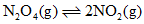At a fixed temperature, the volume of the reaction container is halved. For this change, which of the following statements hold true regarding the equilibrium constant (Kp) and degree of dissociation (α)?

Test: Chemical Equilibrium - 1 - Question 13

Which is correct statement if N2 is added at equilibrium condition?

N2 + 3H2 ⇌ 2NH3

Test: Chemical Equilibrium - 1 - Question 14

The relationship between the equilibrium constant K1 for the reaction.

CO(g) +1/2O2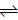​(g) CO2(g)

And the equilibrium constant K2 for the reaction: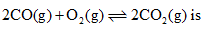Test: Chemical Equilibrium - 1 - Question 15

For the reaction H2 + I2 ⇋ 2HI the equilibrium constant (K) is

Detailed Solution for Test: Chemical Equilibrium - 1 - Question 15

Explanation : aA(g) + bB(g) ----> cC(g) + dD(g)

K = {[C]c[D]d}/{[A]a[B]b}

H2 + I2 ⇋ 2HI

K = {[H2][I2]}/{[2HI]2}

Test: Chemical Equilibrium - 1 - Question 16

For the gas phase reaction.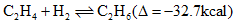Carried out in a vessel, the equilibrium concentration of C2H4 can be increased by
(I) Increasing the temperature
(II) Decreasing the pressure
(III) Removing some H2

*Multiple options can be correct
Test: Chemical Equilibrium - 1 - Question 17

When NaNO3 is heated in a closed vessel, oxygen is liberated and NaNO2 is left behind. At equilibrium.

Detailed Solution for Test: Chemical Equilibrium - 1 - Question 17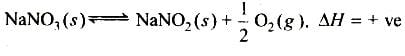Since reaction is endothermic forward reaction is favoured by increase in temperature. Kp = [pO2]1/2.

Thus, addition of NaNO2 or NaNO3 does not cause any change in Kp.

Test: Chemical Equilibrium - 1 - Question 18

The equilibrium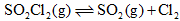is attained at 250C in a closed container and an inert gas, helium is introduced. Which of the following statements are correct?

Test: Chemical Equilibrium - 1 - Question 19

For the reaction,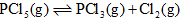The forward reaction at constant temperature is favoured by
(I) Introducing an inert gas at constant volume
(II) Introducing chlorine gas at constant volume
(III) Introducing an inert gas at constant pressure
(IV) Increasing the volume of the container
(V) Introducing PCl5 at constant volume

Detailed Solution for Test: Chemical Equilibrium - 1 - Question 19

According to Le chatelier's principle, if a system in equilibrium is disturbed by changes in determining factors, such as temperature, pressure, and concentration of components then the system will tend to shift its equilibrium position in such a way so as to counteract the effect of the disturbance.

At constant volume, there will be increase in total pressure of the system but the partial pressure of both the reactants and products remain the same. Hence, there will be no effect on equilibrium.

At constant pressure, there will be increase in total volume. Therefore, there will be decrease in number of moles per unit volume of each reactant and product. Hence, the equilibrium will shift towards the side where number of moles are increased.

And if there is an increase in volume of the container, it will directly decrease number of moles per unit volume of each reactant and product, thus, shifting equilibrium towards the side where number of moles are increased.

Test: Chemical Equilibrium - 1 - Question 20

For the reaction,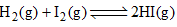the equilibrium constant Kp changes with:

## Physical Chemistry

67 videos|106 docs|31 tests
 Use Code STAYHOME200 and get INR 200 additional OFF Use Coupon Code
Information about Test: Chemical Equilibrium - 1 Page
In this test you can find the Exam questions for Test: Chemical Equilibrium - 1 solved & explained in the simplest way possible. Besides giving Questions and answers for Test: Chemical Equilibrium - 1, EduRev gives you an ample number of Online tests for practice

## Physical Chemistry

67 videos|106 docs|31 tests